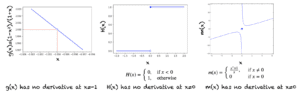# Differentiability and Continuity

For example 3, the function h(x) = 1/x is undefined at the point x=0. Hence, its derivative (-1/x^2) is also not defined at x=0. If a function is not continuous at a point, then it does not have a derivative at that point. Below are a few scenarios, where a function is not differentiable:

1. If the function is not defined at a point
2. Function does not have a limit at that point
3. If the function is not continuous at a point
4. The function has a sudden jump at a point

Following are a few examples:Examples of Points at Which there is no Derivative

## Extensions

This section lists some ideas for extending the tutorial that you may wish to explore.

• Velocity and instantaneous rates of change
• Rules for derivatives
• Integration